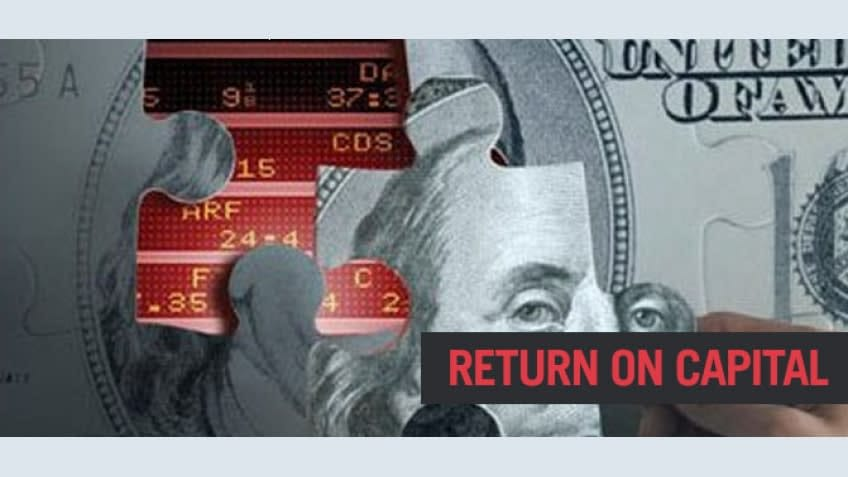## Return on Capital

Return on capital (ROC) is a ratio used in finance, valuation, and accounting. The ratio is estimated by dividing the after-tax operating income (NOPAT) by the book value of invested capital.

Return on capital (ROC), or return on investment (ROI), is one the most important ratios to measure profitability of a company. It measures how much money a business or investment is able to generate on the capital employed. Despite its importance, this ratio is seldom reported and often needs to be calculated. Here is how to determine this ratio from the balance sheet and income statement.

### Calculating Return on Capital

• Find out the net earnings in a given year from the income statement. This is typically located at the bottom line.
• Determine the total capital at the beginning of the given year from the balance sheet. Add up debt and total shareholder equity (which includes preferred stocks, common stocks, and capital surplus and retained earnings).
• Divide net earnings by total capital, which is the return on capital.

Return on capital is a better measure of investment return than return on equity (ROE) or return on assets (ROA). Equity does not account for all the capital a company uses to finance its operations, thus return on equity may seem artificially high for a highly leveraged company using a lot of debt. For example, if you put in \$1000 to start a business, borrow \$10000, and make \$500 after one year, your return on equity is a generous \$500/\$1000, or 50% per annum. This seems too good to be true. Well, it is. The actual return on invested capital is \$500/(\$1000+\$10000) = 4.55%, a more reasonable figure. Return on assets, on the other hand, is unreliable, as the figures for plants and properties are a rough estimate at best (since there is generally no ready market for them), and goodwill and intangibles on the asset account are generally given arbitrary figures.

• The higher the return on capital, the better. The most important thing to look for is consistency. If a company can consistently make 15% or more return on capital over the past 10 years, that is an excellent company. Also compare return on capital with the company's competitors.

Cash flow return on investment is a valuation model that assumes the stock market sets prices based on cash flow, not on corporate performance and earnings.
Cash flow return on investment = Cash Flow/Market Recapitalization
For the corporation, it is essentially internal rate of return (IRR). Cash flow return on investment is compared to a hurdle rate to determine if investment/product is performing adequately. The hurdle rate is the total cost of capital for the corporation calculated by a mix of cost of debt financing plus investors `expected return on equity investments. The Cash flow return on investment must exceed the hurdle rate to satisfy both the debt financing and the investors expected return.
Cash flow return on investment = Gross Cash Flow / Gross Investment
Cash flow return on investment = (Gross Cash Flow - Economic Depreciation) / Gross Investment
This annuity is called the economic depreciation.
Economic Depreciation = (Replacement Cost in Current dollars (Kc)) / ((1+ Kc )^n - 1)
In which n is the expected life of the asset, Kc is cost of capital.

Profit rate: It is the relative profitability of an investment project, of a capitalist enterprise, or of the capitalist economy as a whole. It is similar to the concept of the rate of return on investment. The rate of profit (r) would be measured as
r = (surplus-value)/(capital invested).
The rate of profit depends on the definition of capital invested. Two measurements of the value of capital exist: capital at historical cost and capital at market value. Historical cost is the original cost of an asset at the time of purchase or payment. Market value is the re-sale value, replacement value, or value in present or alternative use.

To compute the rate of profit, replacement cost of capital assets must be used to define the capital cost. Assets such as machinery cannot be replaced at their historical cost but must be purchased at the current market value. When inflation occurs, historical cost would not take account of rising prices of equipment. The rate of profit would be overestimated using lower historical cost for computing the value of capital invested.
On the other side, due to technical progress, products tend to become cheaper. This in itself should raise rates of profit, because replacement cost declines.

The rate of return on a portfolio is "a weighted average of the rates of return on the various assets with the weights being equal to the fractions of the individual's wealth held in these assets" This equates to an average of the average returns on a portfolio taking into account what portion of the portfolio each individual return represents.Eshna